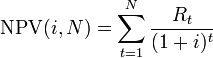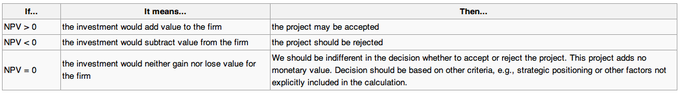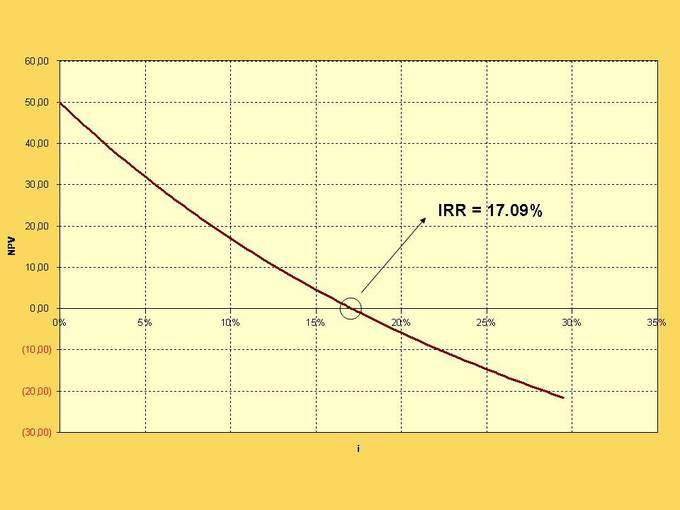Defining NPV

Net Present Value (NPV) is the sum of the present values of the cash inflows and outflows.

Learning Objectives

Define Net Present Value

Key Takeaways

Key Points

• Because of the time value of money, cash inflows and outflows only can be compared at the same point in time.
• NPV discounts each inflow and outflow to the present, and then sums them to see how the value of the inflows compares to the other.
• A positive NPV means the investment is worthwhile, an NPV of 0 means the inflows equal the outflows, and a negative NPV means the investment is not good for the investor.

Key Terms

• cash inflow: Cash that is received by the investor. For example, dividends paid on a stock owned by the investor is a cash inflow.
• cash outflow: Any cash that is spent or invested by the investor.

Every investment includes cash outflows and cash inflows. There is the cash that is required to make the investment and (hopefully) the return.

In order to see whether the cash outflows are less than the cash inflows (i.e., the investment earns a positive return), the investor aggregates the cash flows. Since cash flows occur over a period of time, the investor knows that due to the time value of money, each cash flow has a certain value today. Thus, in order to sum the cash inflows and outflows, each cash flow must be discounted to a common point in time.Airplane: Before purchasing a new airplane, airlines evaluate the NPV of the plan by calculating the PV of the revenue it can earn from it and the PV of its cost (e.g., purchase cost, maintenance, fuel, etc. ).

The net present value (NPV) is simply the sum of the present values (PVs) and all the outflows and inflows:

NPV = PVInflows+ PVOutflows

Don’t forget that inflows and outflows have opposite signs; outflows are negative.

Also recall that PV is found by the formula $\text{PV}=\frac { \text{FV} }{ { (1+\text{i}) }^{ \text{t} } }$ where FV is the future value (size of each cash flow), i is the discount rate, and t is the number of periods between the present and future. The PV of multiple cash flows is simply the sum of the PVs for each cash flow.

The sign of NPV can explain a lot about whether the investment is good or not:

• NPV > 0: The PV of the inflows is greater than the PV of the outflows. The money earned on the investment is worth more today than the costs, therefore, it is a good investment.
• NPV = 0: The PV of the inflows is equal to the PV of the outflows. There is no difference in value between the value of the money earned and the money invested.
• NPV < 0: The PV of the inflows is less than the PV of the outflows. The money earned on the investment is worth less today than the costs, therefore, it is a bad investment.

Calculating the NPV

The NPV is found by summing the present values of each individual cash flow.

Learning Objectives

Calculate a project’s Net Present Value.

Key Takeaways

Key Points

• Cash inflows have a positive sign, while cash outflows are negative.
• To find the NPV accurately, the investor must know the exact size and time of occurrence of each cash flow. This is easy to find for some investments (like bonds ), but more difficult for others (like industrial machinery).
• Investors use different rates for their discount rate such as using the weighted average cost of capital, variable rates, and reinvestment rate.

Key Terms

• discount rate: The interest rate used to discount future cash flows of a financial instrument; the annual interest rate used to decrease the amounts of future cash flow to yield their present value.
• variable: something whose value may be dictated or discovered.
• cash flow: The sum of cash revenues and expenditures over a period of time.

Calculating the NPV

The NPV of an investment is calculated by adding the PVs ( present values ) of all of the cash inflows and outflows. Cash inflows (such as coupon payments or the repayment of principal on a bond) have a positive sign while cash outflows (such as the money used to purchase the investment) have a negative sign.Net Present Value (NPV) Formula: NPV is the sum of of the present values of all cash flows associated with a project. The business will receive regular payments, represented by variable R, for a period of time. This period of time is expressed in variable t. The payments are discounted using a selected interest rate, signified by the i variable.

The accurate calculation of NPV relies on knowing the amount of each cash flow and when each will occur. For securities like bonds, this is an easy requirement to meet. The bond clearly states when each coupon payment will occur, the size of each payment, when the principal will be repaid, and the cost of the bond. For other investments, this is not so simple to determine. When a new piece of machinery is purchased, for example, the investor (the purchasing company) has to estimate the size and occurrence of maintenance costs as well as the size and occurrence of the revenues generated by the machine.

The other integral input variable for calculating NPV is the discount rate. There are many methods for calculating the appropriate discount rate. A firm’s weighted average cost of capital after tax (WACC) is often used. Since many people believe that it is appropriate to use higher discount rates to adjust for risk or other factors, they may choose to use a variable discount rate.

Another approach to selecting the discount rate factor is to decide the rate that the capital needed for the project could return if invested in an alternative venture. If, for example, the capital required for Project A can earn 5% elsewhere, use this discount rate in the NPV calculation to allow a direct comparison to be made between Project A and the alternative. Related to this concept is to use the firm’s reinvestment rate. Reinvestment rate can be defined as the rate of return for the firm’s investments on average, which can also be used as the discount rate.

Interpreting the NPV

A positive NPV means the investment makes sense financially, while the opposite is true for a negative NPV.

Learning Objectives

Interpret a series of net present value calculations

Key Takeaways

Key Points

• When inflows exceed outflows and they are discounted to the present, the NPV is positive. The investment adds value for the investor. The opposite is true when NPV is negative.
• A NPV of 0 means there is no change in value from the investment.
• In theory, investors should invest when the NPV is positive and it has the highest NPV of all available investment options.
• In practice, determining NPV depends on being able to accurately determine the inputs, which is difficult.

Key Terms

• cash flow: The sum of cash revenues and expenditures over a period of time.

The NPV is a metric that is able to determine whether or not an investment opportunity is a smart financial decision. NPV is the present value (PV) of all the cash flows (with inflows being positive cash flows and outflows being negative), which means that the NPV can be considered a formula for revenues minus costs. If NPV is positive, that means that the value of the revenues (cash inflows) is greater than the costs (cash outflows). When revenues are greater than costs, the investor makes a profit. The opposite is true when the NPV is negative. When the NPV is 0, there is no gain or loss.

In theory, an investor should make any investment with a positive NPV, which means the investment is making money. Similarly, an investor should refuse any option that has a negative NPV because it only subtracts from the value. When faced with multiple investment choices, the investor should always choose the option with the highest NPV. This is only true if the option with the highest NPV is not negative. If all the investment options have negative NPVs, none should be undertaken.

The decision is rarely that cut and dry, however. The NPV is only as good as the inputs. The NPV depends on knowing the discount rate, when each cash flow will occur, and the size of each flow. Cash flows may not be guaranteed in size or when they occur, and the discount rate may be hard to determine. Any inaccuracies and the NPV will be affected, too.Machinery: Being able to accurately find the NPV of a piece of machinery means having a good idea when all costs are going to occur (when it will need fixing) and when it will generate revenue (when it will be used on a job).

NPV is easy to use, easily comparable, and customizable.

Learning Objectives

Describe the advantages of using net present value to evaluate potential investments

Key Takeaways

Key Points

• When NPV is positive, it adds value to the firm. When it is negative, it subtracts value. An investor should never undertake a negative NPV project.
• As long as all options are discounted to the same point in time, NPV allows for easy comparison between investment options. The investor should undertake the investment with the highest NPV, provided it is possible.
• An advantage of NPV is that the discount rate can be customized to reflect a number of factors, such as risk in the market.

Key Terms

• gain (or loss): If an investment earns more value than it costs, the difference is the gain. If it costs more than it earns, the difference is a loss.

Calculating the NPV is a way investors determine how attractive a potential investment is. Since it essentially determines the present value of the gain or loss of an investment, it is easy to understand and is a great decision making tool.

When NPV is positive, the investment is worthwhile; On the other hand, when it is negative, it should not be undertaken; and when it is 0, there is no difference in the present values of the cash outflows and inflows. In theory, an investor should undertake positive NPV investments, and never undertake negative NPV investments. Thus, NPV makes the decision making process relatively straight forward.NPV Decision Table: NPV simply and clearly shows whether a project adds value to the firm or not. It’s easy of use in decision making is one of its advantages.

Another advantage of the NPV method is that it allows for easy comparisons of potential investments. As long as the NPV of all options are taken at the same point in time, the investor can compare the magnitude of each option. When presented with the NPVs of multiple options, the investor will simply choose the option with the highest NPV because it will provide the most additional value for the firm. However, if none of the options has a positive NPV, the investor will not choose any of them; none of the investments will add value to the firm, so the firm is better off not investing.

Furthermore, NPV is customizable so that it accurately reflects the financial concerns and demands of the firm. For example, the discount rate can be adjusted to reflect things such as risk, opportunity cost, and changing yield curve premiums on long-term debt.

NPV is hard to estimate accurately, does not fully account for opportunity cost, and does not give a complete picture of an investment’s gain or loss.

Learning Objectives

Discuss the disadvantages of using the NPV method

Key Takeaways

Key Points

• NPV is based on future cash flows and the discount rate, both of which are hard to estimate with 100% accuracy.
• There is an opportunity cost to making an investment which is not built into the NPV calculation.
• Other metrics, such as internal rate of return, are needed to fully determine the gain or loss of an investment.

Key Terms

• Opportunity cost: The cost of an opportunity forgone (and the loss of the benefits that could be received from that opportunity); the most valuable forgone alternative.

There are a number of disadvantages to NPV. NPV is still commonly used, but firms will also use other metrics before making investment decisions.Medicine: Drug developers must try to calculate the future revenues of a drug in order to find the NPV to determine if it is worth the cost of development.

The first disadvantage is that NPV is only as accurate as the inputted information. It requires that the investor know the exact discount rate, the size of each cash flow, and when each cash flow will occur. Often, this is impossible to determine. For example, when developing a new product, such as a new medicine, the NPV is based on estimates of costs and revenues. The cost of developing the drug is unknown and the revenues from the sale of the drug can be hard to estimate, especially many years in the future.

Furthermore, the NPV is only useful for comparing projects at the same time; it does not fully build in opportunity cost. For example, the day after the company makes a decision about which investment to undertake based on NPV, it may discover there is a new option that offers a superior NPV. Thus, investors don’t simply pick the option with the highest NPV; they may pass on all options because they think another, better, option may come along in the future. NPV does not build in the opportunity cost of not having the capital to spend on future investment options.

Another issue with relying on NPV is that it does not provide an overall picture of the gain or loss of executing a certain project. To see a percentage gain relative to the investments for the project, internal rate of return (IRR) or other efficiency measures are used as a complement to NPV.

NPV Profiles

The NPV Profile graphs the relationship between NPV and discount rates.

Learning Objectives

Describe the relationship between a project’s discount rate and its Net Present Value

Key Takeaways

Key Points

• The NPV Profile is a graph with the discount rate on the x-axis and the NPV of the investment on the y-axis.
• Higher discount rates mean cash flows that occur sooner are more influential to NPV. Since the earlier payments tend to be the outflows, the NPV profile generally shows an inverse relationship between the discount rate and NPV.
• The discount rate at which the NPV equals 0 is called the internal rate of return (IRR).

Key Terms

• discount rate: The interest rate used to discount future cash flows of a financial instrument; the annual interest rate used to decrease the amounts of future cash flow to yield their present value.
• internal rate of return: IRR. The rate of return on an investment which causes the net present value of all future cash flows to be zero.

NPV Profiles

The NPV calculation involves discounting all cash flows to the present based on an assumed discount rate. When the discount rate is large, there are larger differences between PV and FV (present and future value) for each cash flow than when the discount rate is small. Thus, when discount rates are large, cash flows further in the future affect NPV less than when the rates are small. Conversely, a low discount rate means that NPV is affected more by the cash flows that occur further in the future.

The relationship between NPV and the discount rate used is calculated in a chart called an NPV Profile. The independent variable is the discount rate and the dependent is the NPV. The NPV Profile assumes that all cash flows are discounted at the same rate.NPV Profile: The NPV Profile graphs how NPV changes as the discount rate used changes.

The NPV profile usually shows an inverse relationship between the discount rate and the NPV. While this is not necessarily true for all investments, it can happen because outflows generally occur before the inflows. A higher discount rate places more emphasis on earlier cash flows, which are generally the outflows. When the value of the outflows is greater than the inflows, the NPV is negative.

A special discount rate is highlighted in the IRR, which stands for Internal Rate of Return. It is the discount rate at which the NPV is equal to zero. And it is the discount rate at which the value of the cash inflows equals the value of the cash outflows.